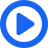If you're seeing this message, it means we're having trouble loading external resources on our website.

If you're behind a web filter, please make sure that the domains *.kastatic.org and *.kasandbox.org are unblocked.

# Thin lens equation and problem solving

Some examples of using the thin lens equation. Created by David SantoPietro.

## Want to join the conversation?

• Why does the object distance have to be a positive? Do we not follow the Cartesian sign conventions for thin lenses?•I don't actually think he is using Cartesian convention; I think under Cartesian convention, anything to the left of the lens, say, is negative, and anything to the opposite side is positive. So for a convex lens, u (on the left) would be negative and v (to the right) would be positive.
• At how did 1/-8cm minus 1/24cm come out to be 1/6cm?• I think something is wrong..Object distances are always negative aacording to modern cartesian sign system./.!!• At can't it be explained like.. if the image is real the image distance is positive and if the image is virtual the image distance is negative? :-)• At the image was described to be as positive. Using Sal's equation in an earlier video, we have that (do/di) = (ho/hi). In a hypothetical example, let's assume the height of the original image is 8 cm. Using the rest of the values from the video, we get (24/-6) = (8/x). Solving for x yields -2, which means that the image is inverted. Although the magnitude is correct, according to this video, the number should be positive. Why am I getting a negative number using Sal's equation as opposed to the Magnification equation?• I thought concave focal length is always positive and convex is always negative? that's what my physics book says and what we've been taught...• i thought the lens formula is ...
1/v-1/u=1/f

where u = object distance
v = image distance
f = focal length.• If you did this problem using the equation 1/f=1/v-1/u, you would get the answer as 6 cm.
According to the same sign convention using which the above mentioned formula was derived, the answer 6 cm means the same as -6 cm when viewed from different sign conventions.
The sign convention used deriving the above mentioned formula is known as the Cartesian sign convention. According to this, imagine a lens placed such that its optical centre coincides with the origin of the Cartesian plane. In a Cartesian plane rightwards and upwards are considered to be positive directions, whereas, leftwards and downwards are considered to be the negative directions. So, the same thing is applicable to lenses. So its always imagined that light rays are passed through the lens in the rightward direction.
Hope this helps!
(1 vote)
• In the video, there was no convex lens example, it just said that the focal length is positive. can someone plz give me an example of it (and the solution), because it is very confusing.• In this case, the focal length of the convex lens' focal length would be positive, totally opposite with the Cartesian sign convention, If you apply this method then you should be careful not to be confused.
Both method are correct and the discrepancies are base on where is your point of view, though Cartesian sign convention is being use more widely.
(1 vote)
• Hi, Watch from`3:25 to3:30`, Sal is describing about the object distance but I think that the object distance from every lens would be negative every time, weather it is a concave or convex, Right! Can any one tell me Whether I am Right or wrong.